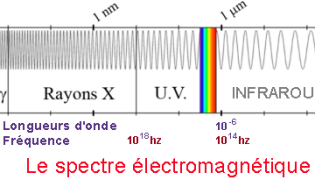# Light and energy

## The photon energy

Category: light and photons
Updated June 01, 2013

Sunlight heats the Earth. This energy comes from the electromagnetic waves and in particular photons that strike the surface of our planet. The light is part of a small range of electromagnetic vibrations found in the electromagnetic spectrum. The Earth's atmosphere allows only a tiny fraction of this radiation.
Shortwave is absorbed in the atmospheric layers and long waves are reflected. The greater part waves reaching us, are those of visible light. The nature of light is the quantum mechanics for which it is both a wave and a particle. The light has a wavelength, which determines the color, the Red emits in the wavelength from 620 to 780 nm, the Orange from 592 to 620 nm, the yellow from 578 to 592 nm, Green from 500 to 578 nm, the blue from 446 to 600 nm and Violet 380 to 446 nm. The various windows of the electromagnetic spectrum are characterized by a wavelength, but also by a defined frequency range. The frequency is the number of electromagnetic oscillations which pass through a given point in one second. It is expressed with the unit of frequency what is the hertz. More the wavelength is shorter, the frequency is high.

When we receive the light rays of the sun on our skin, we are well aware that photons carry energy.
But how much energy a photon carries?
It depends on the photon or rather it depends on the wavelength of the photon. Over the wavelength of the photon is shorter and it is energy.
This is the formula E = hf which gives a photon energy.
E is the energy expressed in Joules.
h is Planck's constant (6.62 x 10-34).
f is the frequency. The energy of a photon is infinitely small.

nota: Light is the primary source of energy emitted by the Sun. It supplies terrestrial ecosystems via photosynthesis.

### Image: The electromagnetic spectrum includes all the windows of light. Maxwell determined that light is an electromagnetic wave and there is no reason to limit the wavelength thereof to the interval corresponding to the spectrum of visible light, full spectrum is light.## How many photons does it take to heat a cup of coffee?

It depends on the frequency, so on the color.
The frequency of red is 350 THz and that of blue 750 THz.
The formula E = hf gives the energy of a photon.
Energy of a blue photon:
E=6.62x10-34x750x1012 ≈ 10-18 joule.
1 joule ≈ 1018 blue photons.
The calorie is the amount of energy required to raise the temperature of a gram of liquid water from 14.5 to 15.5 ° C. This corresponds to 4.1855 joules.
To raise the temperature of 10 cm3 of coffee by 50 °C, approximately 100x50x1018 or 1021 or 1021 blue photons and 1022 red photons.
The shorter the wavelength, the greater the energy of the radiation.

nota:
1 kJ is approximately 239 calories.
1 Ws (Watt seconds) = 1 joule.
1 kWh (kilowatt hours) = 3,600,000 Joules.

### Image: spectrum of visible light ranging from the infrared to ultraviolet, corresponds to the wavelengths of 400 nanometers in the violet to 800 nanometers in the red, i.e. 4x10-7 to 8x10-7 m. Between the wavelength (λ) and the frequency (ν) the following relationship: ν = c / λ where c is the speed of light is about 300 000 km/s.## What is the solar power received by the Earth?

The mean temperature at Earth is related to the average solar power received on Earth and Earth / Sun distance.
The calculation of the received power on Earth in watts per square meter (see image opposite) is given by:
Pr = x L/4ΠR2 Πr2
Calculating the power absorbed by the Earth (the cons) is given by Pa = Pr (1-A)
L/4ΠR2 is the solar constant, it equals 1368 wm2.
The average value (there is a hemisphere that receives the radiation) is 342 wm2 given albedo it is 239 wm2.
But the Earth re-emits light in space.
Earth is in thermodynamic equilibrium, i.e. that the power absorbed is equal to the power re-emitted.
Calculating the mean temperature of the Earth gives 255 K, but the greenhouse effect produced by the presence of water vapor and carbon dioxide in the atmosphere of the Earth, increasing the temperature of 33 K, it is 288 K is therefore about 15 ° C.### Image: Calculating the average power received by the Earth, University Pierre et Mari.e. Curie, Paris VI### Image: Calculating the average power received by the Earth, University Pierre et Mari.e. Curie, Paris VI

 1997 © Astronoo.com − Astronomy, Astrophysics, Evolution and Earth science. Contact    Mentions légales